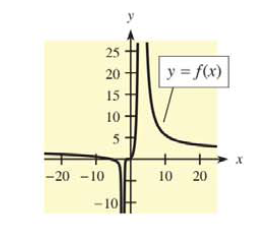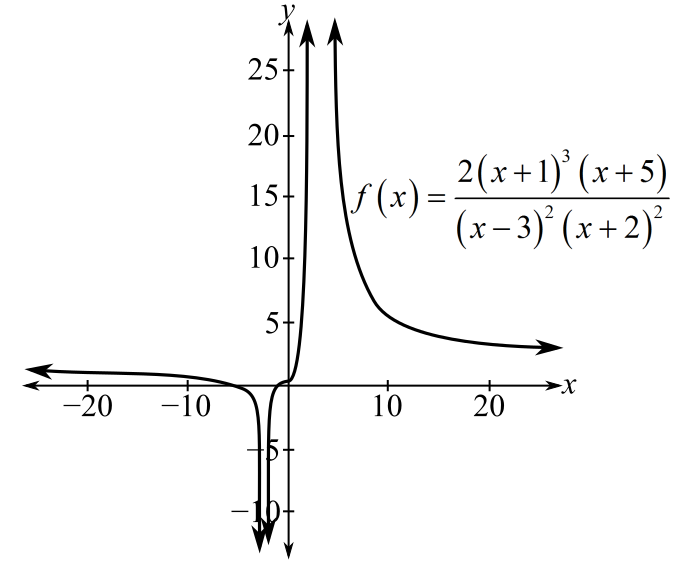Chapter 9.2, Problem 23E### Mathematical Applications for the ...

11th Edition
Ronald J. Harshbarger + 1 other
ISBN: 9781305108042

#### Solutions

Chapter
Section### Mathematical Applications for the ...

11th Edition
Ronald J. Harshbarger + 1 other
ISBN: 9781305108042
Textbook Problem

# Each of Problems 21-24 contains a function and its graph. For each problem, answer parts (a) and (b).(a) Use the graph to determine, as well as you can,(i) vertical asymptotes, (ii) lim lim x → ∞   f ( x ) .(iii) lim x →   −   ∞   f ( x ) . (iv) horizontal asymptotes.(b) Check your conclusions in (a) by using the functions to determine items (i)-(iv) analytically f ( x ) = 2 ( x + 1 ) 3 ( x + 5 ) ( x − 3 ) 2 ( x + 2 ) 2(a)

To determine

The vertical asymptote of the function f(x)=2(x+1)3(x+5)(x3)2(x+2)2, limxf(x), limxf(x) and horizontal asymptotes using the graph of the function,Explanation

Given Information:

The function is f(x)=2(x+1)3(x+5)(x3)2(x+2)2. The graph for the function is

Explanation:

Consider the provided function,

f(x)=2(x+1)3(x+5)(x3)2(x+2)2

Consider the provided graph of the function,

The vertical asymptote is the line, which is approached by the graph of the function, but never really intersects or touches it.

In the provided graph, the lines x=2 and x=3 are the lines which are approached by the graph of the function, but never really intersects or touches it

(b)

To determine

Whether the vertical asymptote of the function f(x)=2(x+1)3(x+5)(x3)2(x+2)2, limxf(x), limxf(x) and horizontal asymptotes calculated using the graph of the function is correct or not,### Still sussing out bartleby?

Check out a sample textbook solution.

See a sample solution

#### The Solution to Your Study Problems

Bartleby provides explanations to thousands of textbook problems written by our experts, many with advanced degrees!

Get Started